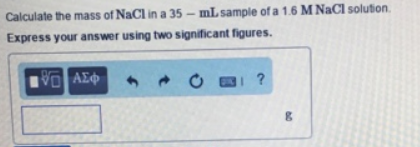# Problem: Calculate the mass of NaCl in a 35-mL sample of a 1.6 M NaCl solution. Express your answer using two significant figures.

###### FREE Expert Solution
93% (341 ratings)###### Problem Details

Calculate the mass of NaCl in a 35-mL sample of a 1.6 M NaCl solution. Express your answer using two significant figures.